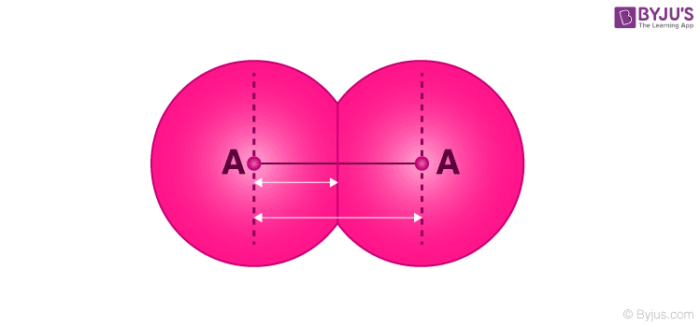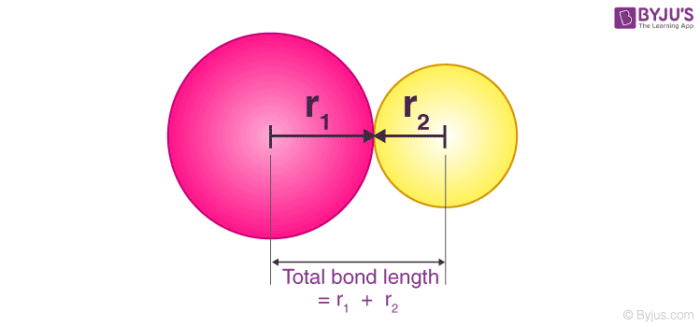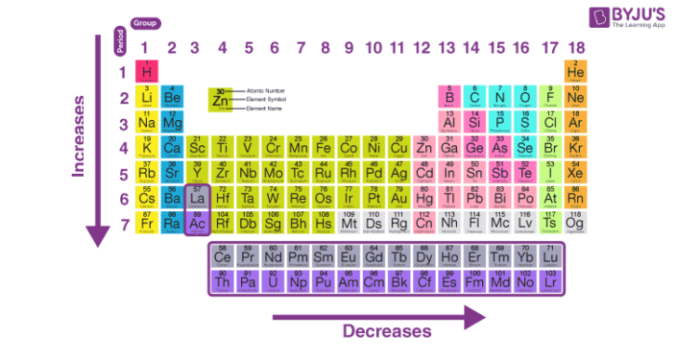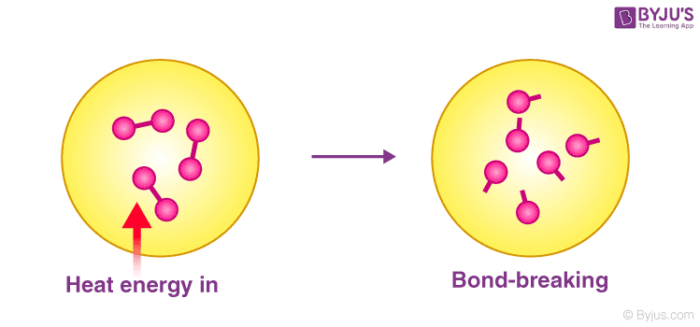Checkout JEE MAINS 2022 Question Paper Analysis : Checkout JEE MAINS 2022 Question Paper Analysis :

# Bond Parameters

## What are Bond Parameters?

Covalent bonds can be characterized on the basis of several bond parameters such as bond length, bond angle, bond order, and bond energy (also known as bond enthalpy). These bond parameters offer insight into the stability of a chemical compound and the strength of the chemical bonds holding its atoms together.## Bond Order

The bond order of a covalent bond is the total number of covalently bonded electron pairs between two atoms in a molecule. It can be found by drawing the Lewis structure of the molecule and counting the total number of electron pairs between the atoms in question.

• Single bonds have a bond order of 1.
• Double bonds have a bond order of 2.
• Triple bonds have a bond order of 3.

Note: If the bond order of a covalent bond is 0, the two atoms in question are not covalently bonded (no bond exists).

### Examples

• The bond order of the carbon-hydrogen bond in C2H2 (ethyne/acetylene) is 1 and that of the carbon-carbon bond is 3.
• The bond order of the oxygen-oxygen bond in an O2 molecule is 2.
• In a carbon monoxide molecule, the carbon-oxygen bond has a bond order of 3, as illustrated in the Lewis structure provided below.• Since the nitrate ion is stabilized by resonance, the bond order of the nitrogen-oxygen bond is 4/3 or 1.33. It is calculated by dividing the total number of nitrogen-oxygen bonds (4) by the total number of covalently bonded nitrogen-oxygen groups (3).

### Bond Order as per the Molecular Orbital Theory

As per the molecular orbital theory, the bond order of a covalent bond is equal to half of the difference between the number of bonding and anti bonding electrons, as represented by the following formula:

Bond Order = (½)*(total no. of bonding electrons – total no. of anti bonding electrons)

## Bond Angle

Bond angle can be defined as the angle formed between two covalent bonds that originate from the same atom. An illustration detailing the bond angle in a water molecule (104.5oC) is provided below.The geometric angle between any two adjacent covalent bonds is called a bond angle. This bond parameter provides insight into the molecular geometry of a compound.

## Bond Length

Bond length is a measure of the distance between the nuclei of two chemically bonded atoms in a molecule. It is approximately equal to the sum of the covalent radii of the two bonded atoms. For covalent bonds, the bond length is inversely proportional to the bond order – higher bond orders result in stronger bonds, which are accompanied by stronger forces of attraction holding the atoms together. Short bonds are a consequence of these strong forces of attraction.An illustration describing the bond length of a covalent bond in terms of the sum of the individual covalent radii of the participating atoms is provided above. This bond parameter can be experimentally determined via the following techniques:

• Rotational spectroscopy.
• X-ray diffraction.
• Neutron diffraction.

Bonded atoms tend to absorb thermal energy from their surroundings and constantly vibrate. This vibration causes the bond length to vary. Therefore, it is important to note that the bond length of a covalent bond represents the average distance between the nuclei of the participating atoms.

### Periodic Trends in Bond Length

Bond lengths are directly proportional to the atomic radii of the participating atoms. The periodic trends that can be observed in the bond lengths of elements are similar to the periodic trends in the atomic radii of the elements (decreases across the period, increases down the group).An illustration detailing the periodic trends in bond length is provided above. It can be noted that the H-H bond is the bond with the shortest bond length (74 picometers).

## Bond Energy or Bond Enthalpy

Bond energy is a measure of the strength of a chemical bond. It can be defined as the energy required to break all covalent bonds of a specific type in one mole of a chemical compound (which is in its gaseous state).It is important to note that bond energy is not the same as bond dissociation energy. The latter is the change in enthalpy associated with the homolytic cleavage of a bond whereas the former is the average of the bond dissociation enthalpies of all bonds (of a specific type) in a molecule.

### Factors Affecting Bond Energy

The strength of a chemical bond is directly proportional to the amount of energy required to break it. Therefore, bond energy is:

• Inversely proportional to the bond length, i.e. longer bonds have lower bond energies.
• Directly proportional to the bond order, i.e. multiple bonds have high bond energies.
• Inversely proportional to the atomic radii of the atoms participating in the bond (since the atomic radius is directly proportional to bond length).

Note: the difference in the electronegativities of the atoms participating in the chemical bond also contributes to the bond energy.

## Frequently Asked Questions – FAQs

### What is mean by bond angle?

The angle formed between three atoms over at least two bonds is a bond angle. The angle of torsion for the four atoms bonded together in a chain is the angle between the plane created by the first three atoms and the plane created by the last three atoms.

### Which bond is the strongest bond?

Thus, we find that triple bonds between the same two atoms are stronger and shorter than double bonds; similarly, double bonds between the same two atoms are stronger and shorter than single bonds.

### Is a double bond or triple bond stronger?

Owing to the presence of two pi bonds, rather than one, triple bonds are stronger than double bonds. Each carbon has two hybrid sp-orbitals and one of them overlaps to form a sp-sp sigma bond with the matching one from the other carbon atom.

### Are polar or nonpolar bonds stronger?

One may assume that due to the coulomb forces involved, polar bonds are stronger, but they are soluble in water, etc., so that the bond can be quickly dissolved, whereas nonpolar bonds can not be dissolved so quickly even if the forces in play are much weaker.

### What is enthalpy of bond dissociation?

The amount of energy contained in a bond between atoms in a molecule is defined by bond enthalpy, which is also known as bond-dissociation enthalpy, average bond energy, or bond strength. In fact, in the gas phase, it is the energy that needs to be applied to the homolytic or symmetrical cleavage of a bond.

Test your knowledge on bond parameters

1. Ok good super excellent outstanding

2. Abhinitha

Thanks you for this explanation . It helped me a lot.

3. can understand easily thanks for nice information

4. Navaneeth

better than all websites

5. worth of plenty thanks, really helpful a lot

6. Minha Shereefa

Very Thanks for this brief explanation

7. Kahkashan

It really helped in to under the section of bond parameters very much well 😉